### SQL 结构化查询语言

1.相关内容： 在SQL Server 2000中，如果数据库的排序规则为Chinese_PRC_CI_AS，那么查询时是不分大小写的，例如下列这二条SQL语句，查询的结果是一样的。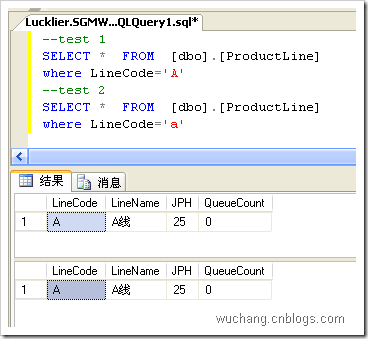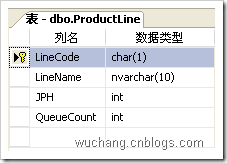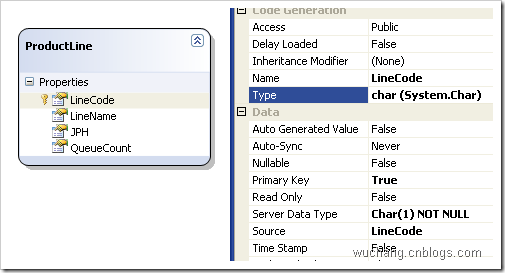表定义 生成的实体

2.

 var test1 = from p in db.ProductLines where p.LineCode =='A' select p;

 SELECT [t0].[LineCode], [t0].[LineName], [t0].[JPH], [t0].[QueueCount] FROM [dbo].[ProductLine] AS [t0] WHERE UNICODE([t0].[LineCode]) = @p0 -- @p0: Input Int (Size = 0; Prec = 0; Scale = 0)  -- Context: SqlProvider(Sql2000) Model: AttributedMetaModel Build: 3.5.21022.8

Linq 语句
 var test1 = from p in db.ProductLines where p.LineCode =='a' select p;
 var test1 = from p in db.ProductLines where p.LineCode =='A' select p;

 SELECT [t0].[LineCode], [t0].[LineName], [t0].[JPH], [t0].[QueueCount] FROM [dbo].[ProductLine] AS [t0] WHERE UNICODE([t0].[LineCode]) = @p0 -- @p0: Input Int (Size = 0; Prec = 0; Scale = 0) 
 SELECT [t0].[LineCode], [t0].[LineName], [t0].[JPH], [t0].[QueueCount] FROM [dbo].[ProductLine] AS [t0] WHERE UNICODE([t0].[LineCode]) = @p0 -- @p0: Input Int (Size = 0; Prec = 0; Scale = 0)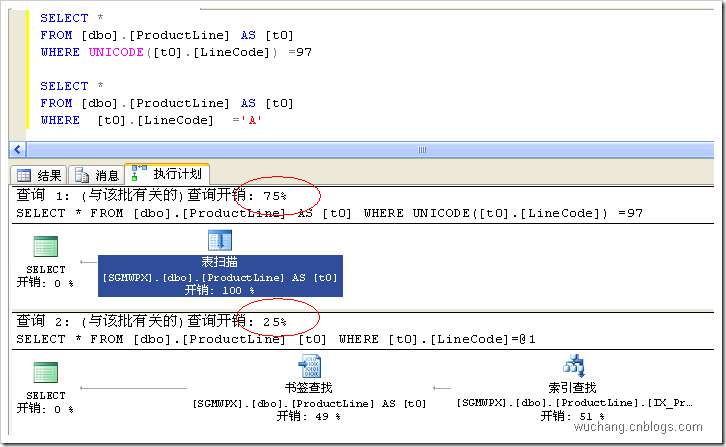3.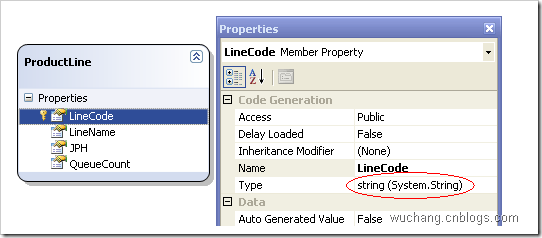var test1 = from p in db.ProductLines where p.LineCode == "a" select p;
 SELECT [t0].[LineCode], [t0].[LineName], [t0].[JPH], [t0].[QueueCount] FROM [dbo].[ProductLine] AS [t0] WHERE [t0].[LineCode] = @p0 -- @p0: Input VarChar (Size = 1; Prec = 0; Scale = 0) [a] -- Context: SqlProvider(Sql2000) Model: AttributedMetaModel Build: 3.5.21022.8
Linq sql

LINQ to SQL:处理char(1)字段的方式会引起全表扫描问题
SQL语句中含有乘号报错的处理办法
SQL中函数 replace 的参数1的数据类型ntext无效的解决方法
SQL附加数据库失败问题的解决方法
sql 数据库出现“只读”提示 解决方法 (sql 错误 5120)
10种Java开发者编写SQL语句时常见错误
SQL Agent服务无法启动的解决方法

sqlserver 因为选定的用户拥有对象，所以无法除去该用户的解决方法

yhdrk 发布于2016-06-08 23:27:43